# Circuits Question: Thevenin Equivalent

• Engineering

## Homework Statement

Find thevenin equivelent of this circuit with respect to a and b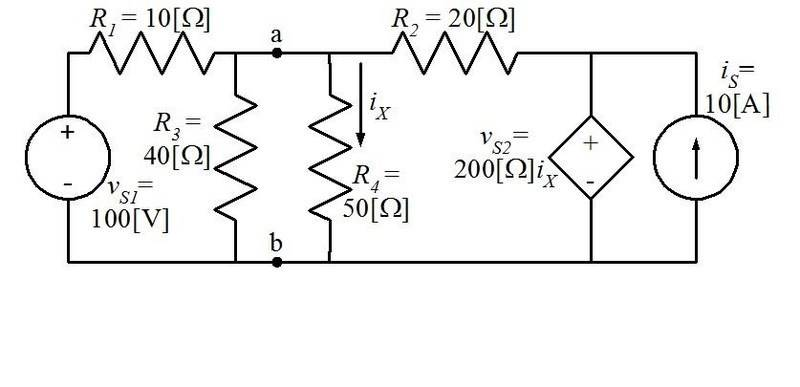Mesh Currents

## The Attempt at a Solution

Here I will try to find V(open circuit) = Vth = Vab ((I think this anyways))

I apply mesh currents (any faster methods are welcome if you know them)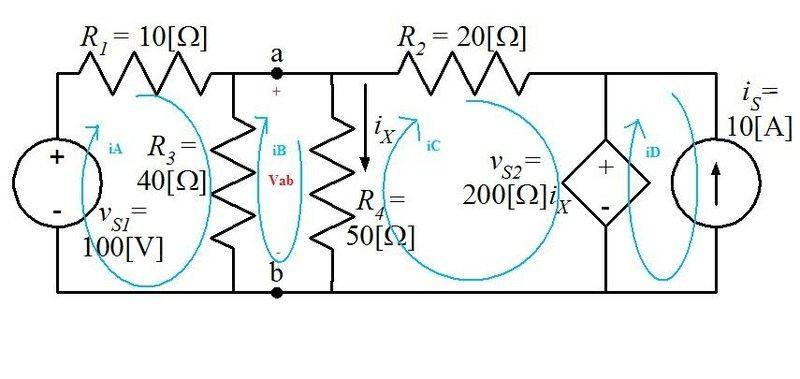iA: -100/V/ + 10/ohm/(iA) + 40/ohm/(iA-iB)=0
iB: 40/ohm/(iB-iA) + 50/ohm/(iB-iC)=0
iC: 50/ohm/(iC-iB) + 20/ohm/(iC) + 200/ohm/(Ix) = 0
iD:-10/A/
iX: iX=iB-Ic

I get the folowing values using solve function on calculator:
iX=-40A/
iA=210/A/
iB=260/A/
iC=300/A/
iD=-10/A/

And using ohms law with parallel resistors having equal voltages..
Vab = 40/ohm/*(iA-iB) = -2000 = Voc = Vth

Now is where I begin to have trouble finding Isc...

I draw the short circuit between a and b (which I believe causes R3 and R4 to have no current through them thus making Ix = 0 and vs2 = 0 as well):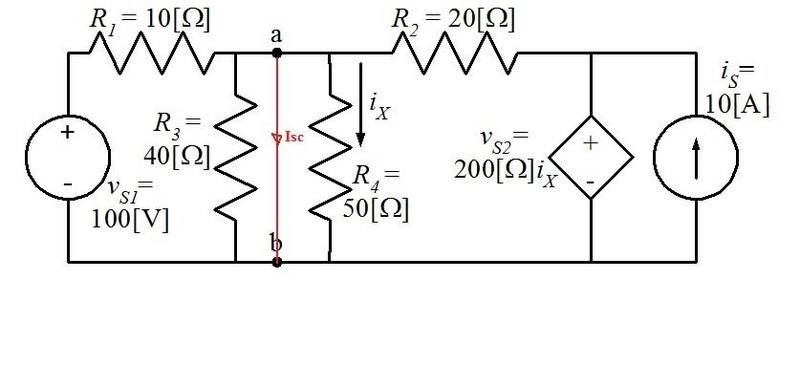Simplifying it you gain ((if my previous assumptions are correct reguarding the short circuit)):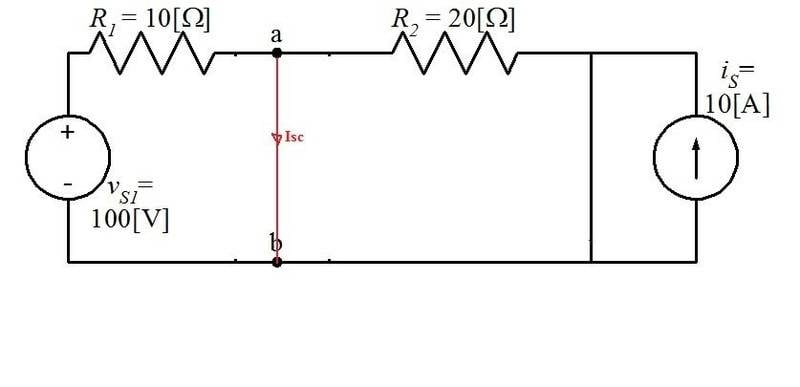Now from here I am actually unsure what I should do. I do want to do mesh currents but I don't know if I will receive a correct answer. I also wondered if I could use a closed surface... something like this perhaps: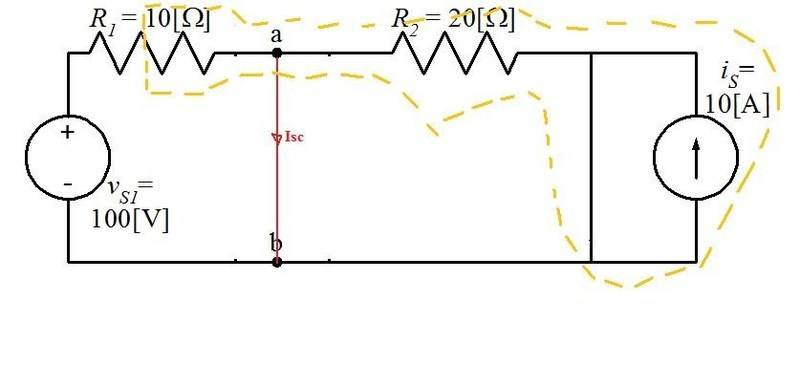If I used that I would get an equation like -100/V/ / 10/Ohms/ + Isc = 0

But then again it just doesn't seem quiet right to me. And i have no way to check my answer.

You could get rid of the 10 amp source (Is) right from the beginning; it does nothing to the circuit to the left of Vs2. It just adds 10 amps to the current in Vs2, which has no effect on Vs2's voltage.

So, Is contributes nothing to the current in the short you've placed across a-b because Vs2 absorbs the entire 10 amps from Is, and the current in the a-b short is just 10 amps.